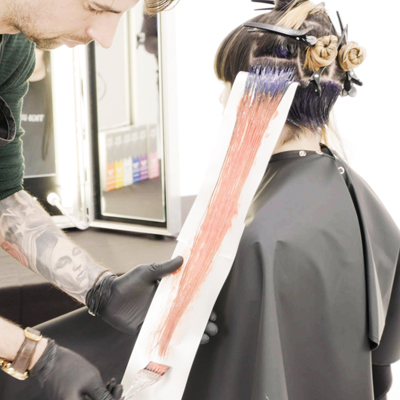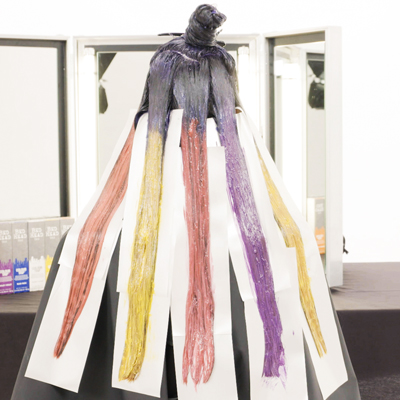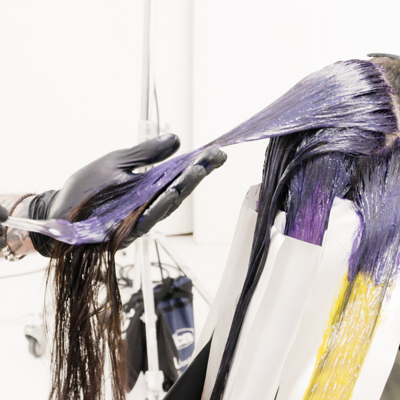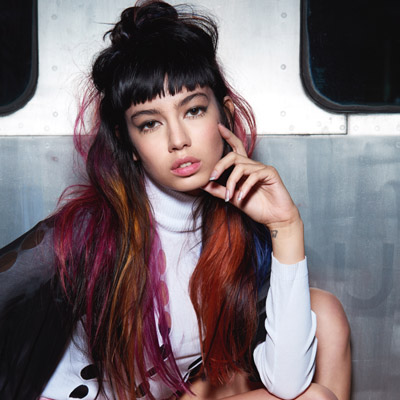How-To: Ribbon Pop
Last updated: May 31, 2017

# How-To: Ribbon Pop

ALL PHOTOS SLIDESHOW
GET THE HOW-TO• Formula A

• Formula B

30g Bed Head by TIGI® Colour Trip Blue + 20g Violet

• Formula C

30g Clear + 10g Pale Yellow + 10g Red + 3g Pink

• Formula D

40g Yellow + 30g Pale Yellow

• Formula E

36g Pale Yellow + 6g Pink + 2g Red

• Formula F

30g Pale Violet + 30g Violet + 1g Blue# How-To: Ribbon Pop

Push the boundaries of color with the new Bed Head Colour Trip semi-permanent line from TIGI®! This look focuses on a high-shine root area with a spectrum of colors throughout.

Manufacturer: TIGI, @tigiprofessionals

## COLOR FORMULAS• Formula A

• Formula B

30g Bed Head by TIGI® Colour Trip Blue + 20g Violet

• Formula C

30g Clear + 10g Pale Yellow + 10g Red + 3g Pink

• Formula D

40g Yellow + 30g Pale Yellow

• Formula E

36g Pale Yellow + 6g Pink + 2g Red

• Formula F

30g Pale Violet + 30g Violet + 1g Blue

## HOW-TO STEPS•• Formula A

• Formula B

30g Bed Head by TIGI® Colour Trip Blue + 20g Violet

• Formula C

30g Clear + 10g Pale Yellow + 10g Red + 3g Pink

• Formula D

40g Yellow + 30g Pale Yellow

• Formula E

36g Pale Yellow + 6g Pink + 2g Red

• Formula F

30g Pale Violet + 30g Violet + 1g Blue

1

To achieve a lighter canvas, apply Formula A 3 inches away from the hairline through the midlengths and ends.

•• Formula A

• Formula B

30g Bed Head by TIGI® Colour Trip Blue + 20g Violet

• Formula C

30g Clear + 10g Pale Yellow + 10g Red + 3g Pink

• Formula D

40g Yellow + 30g Pale Yellow

• Formula E

36g Pale Yellow + 6g Pink + 2g Red

• Formula F

30g Pale Violet + 30g Violet + 1g Blue

2

Begin by taking an irregular shape behind the fringe area, finishing at the top crown.

•• Formula A

• Formula B

30g Bed Head by TIGI® Colour Trip Blue + 20g Violet

• Formula C

30g Clear + 10g Pale Yellow + 10g Red + 3g Pink

• Formula D

40g Yellow + 30g Pale Yellow

• Formula E

36g Pale Yellow + 6g Pink + 2g Red

• Formula F

30g Pale Violet + 30g Violet + 1g Blue

3

To continue, create five sections underneath the top crown, giving you a total of six sections. Leave the front hairline, including the fringe, unclipped.

•• Formula A

• Formula B

30g Bed Head by TIGI® Colour Trip Blue + 20g Violet

• Formula C

30g Clear + 10g Pale Yellow + 10g Red + 3g Pink

• Formula D

40g Yellow + 30g Pale Yellow

• Formula E

36g Pale Yellow + 6g Pink + 2g Red

• Formula F

30g Pale Violet + 30g Violet + 1g Blue

4

Begin at the nape area and apply Formula B to the entire undercut section.

•• Formula A

• Formula B

30g Bed Head by TIGI® Colour Trip Blue + 20g Violet

• Formula C

30g Clear + 10g Pale Yellow + 10g Red + 3g Pink

• Formula D

40g Yellow + 30g Pale Yellow

• Formula E

36g Pale Yellow + 6g Pink + 2g Red

• Formula F

30g Pale Violet + 30g Violet + 1g Blue

5

Progressing onto the side, apply Formula B to the new growth, and use a freehand blending technique to apply Formula C to the lengths and ends.

•• Formula A

• Formula B

30g Bed Head by TIGI® Colour Trip Blue + 20g Violet

• Formula C

30g Clear + 10g Pale Yellow + 10g Red + 3g Pink

• Formula D

40g Yellow + 30g Pale Yellow

• Formula E

36g Pale Yellow + 6g Pink + 2g Red

• Formula F

30g Pale Violet + 30g Violet + 1g Blue

6

Continue working in the same way through the remaining sections, applying Formula B to the new growth, then alternating between Formulas D, E and F, then D again.

•• Formula A

• Formula B

30g Bed Head by TIGI® Colour Trip Blue + 20g Violet

• Formula C

30g Clear + 10g Pale Yellow + 10g Red + 3g Pink

• Formula D

40g Yellow + 30g Pale Yellow

• Formula E

36g Pale Yellow + 6g Pink + 2g Red

• Formula F

30g Pale Violet + 30g Violet + 1g Blue

7

Proceed onto the top section and the front hairline. Apply Formula B from the new growth through the midlengths and ends. Continue until the section is complete.

•• Formula A

• Formula B

30g Bed Head by TIGI® Colour Trip Blue + 20g Violet

• Formula C

30g Clear + 10g Pale Yellow + 10g Red + 3g Pink

• Formula D

40g Yellow + 30g Pale Yellow

• Formula E

36g Pale Yellow + 6g Pink + 2g Red

• Formula F

30g Pale Violet + 30g Violet + 1g Blue

8

Process for up to 30 minutes. Emulsify, rinse and remove using the Bed Head Colour Goddess range.

•• Formula A

• Formula B

30g Bed Head by TIGI® Colour Trip Blue + 20g Violet

• Formula C

30g Clear + 10g Pale Yellow + 10g Red + 3g Pink

• Formula D

40g Yellow + 30g Pale Yellow

• Formula E

36g Pale Yellow + 6g Pink + 2g Red

• Formula F

30g Pale Violet + 30g Violet + 1g Blue

9